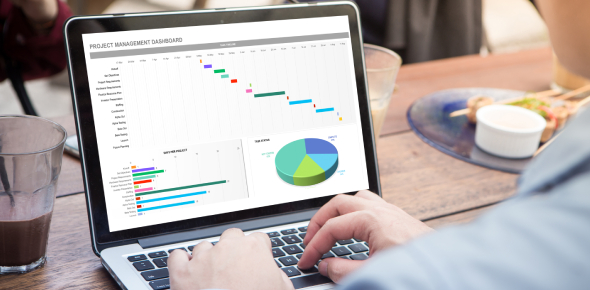# MS Excel Fundamentals Quiz! Trivia

25 Questions | Total Attempts: 34SettingsCreate your own QuizM. S. Excel is an essential application by Microsoft that enables one to present different data types with ease. Do you know how to enter formulas into the system or use the various tools? Can you change cells into rows? This quiz is an excellent place to test out your skills, do give it a shot and see how well you will do!

• 1.
These are rows and columns of data.
• A.

Cells

• B.

Microsoft Excel

• C.

• D.

Workbook

• 2.
These are documents or files created from Microsoft Excel.
• A.

Data

• B.

• C.

Workbooks

• D.

Worksheets

• 3.
It is the cell reference that displays the address of an active cell.
• A.

Formula bar

• B.

Name box

• C.

Range

• D.

Worksheet tab

• 4.
It is a part of the MS Excel environment that is used to displays the content of the selected cell and it is located to the area near the top of the worksheet.
• A.

Formula bar

• B.

Name box

• C.

Status bar

• D.

Worksheet tab

• 5.
The command that you should use if you want to duplicate your worksheet data to other parts of the worksheet area.
• A.

Move and paste

• B.

Copy and paste

• C.

Cut and paste

• D.

Cut and copy

• 6.
The intersection of rows and columns of spreadsheet.
• A.

Range of cells

• B.

Cell

• C.

• D.

Merge cells

• 7.
The spreadsheet command that you should use to add one or more blank rows or columns in an already constructed worksheet.
• A.

Copy

• B.

Delete

• C.

Insert

• D.

Move

• 8.
The symbol that you need to use before you type the formula in a cell.
• A.

+ (plus sign)

• B.

= (equal sign)

• C.

– (minus sign)

• D.

/ (slash)

• 9.
####### in the cell it means that
• A.

Your formula has a syntax error

• B.

The column is too narrow to show all the digits of the number

• C.

The row is too short to show the number at the current font size

• D.

Either b or c

• 10.
The part of MS Excel environment that is used to display the name of the program and the name of the workbook you are currently working on.
• A.

Name box

• B.

Ribbon

• C.

Status bar

• D.

Title bar

• 11.
Keystroke is used in saving a worksheet.
• A.

ALT + S

• B.

CTRL + S

• C.

Shift + A

• D.

Shift + S

• 12.
Data type which contains any combination of letters, numbers, and special characters that are not used in the calculation.
• A.

Date/Time

• B.

Formula

• C.

Label

• D.

Values

• 13.
These data entries are numbers that are can be used in calculation.
• A.

Date/Time

• B.

Formula

• C.

Label

• D.

Values

• 14.
Which of the following buttons are you going to select to stretch Cell C1 so it covers 10 cells?
• A.
• B.
• C.
• D.
• 15.
Text controls that are used to format the horizontal and vertical alignments of data within a cell.
• A.

Merge Cells

• B.

Text Alignment

• C.

Shrink to Fit

• D.

Wrap Text

• 16.
These are spreadsheet pages with in a workbook.
• A.

Cells

• B.

Tables

• C.

Tabs

• D.

Worksheets

• 17.
It is a small black square in the lower-right corner of the cell that you need to drag across in order to automatically copy the cell content in adjacent rows or columns.
• A.

Auto-fill Options

• B.

Border

• C.

Cursor

• D.

Fill handle

• 18.
Column Headers  is to letters as Row Headers is to:
• A.

Formulas

• B.

Numbers

• C.

• D.

Symbols

• 19.
button  represents
• A.

Auto-fill button

• B.

Alphanumeric button

• C.

Sort ascending button

• D.

Sort descending button

• 20.
It is a part of the MS Excel environment that shows the number of worksheets in a workbook.
• A.

Formula bar

• B.

Name box

• C.

Status bar

• D.

Worksheet tab

• 21.
It is the process of placing and organizing records or data in a specified order.
• A.

Auto-fill

• B.

Creating Chart

• C.

Formatting

• D.

Sorting

• 22.
It is the most appropriate formula you can put in the B2 cell to calculate a 7% tax
• A.

=A2*0.07

• B.

=A2*0.7%

• C.

=A2*0.7

• D.

=A2+(A2*0.07)

• 23.
button means:
• A.

Align the text on the borders of the page.

• B.

Extend the content on the entire length of the cell.

• C.

Group together the selected cells and align center the content.

• D.

None of the above

• 24.
Keys that are used to select multiple ranges of adjacent cells.
• A.

ALT

• B.

CTRL

• C.

SHIFT

• D.

TAB

• 25.
What will happen to the value of a cell that contains a number if you drag its fill-handle downward and you select the fill-series option of auto-fill?
• A.

Nothing will happen

• B.

The value will decline into cells that are dragged across.

• C.

The value will be copied into cells that are dragged across.

• D.

The value will increment into cells that are dragged across.

Related TopicsBack to top
×

Wait!
Here's an interesting quiz for you.